[BACK]Computer Modeling in Engineering & SciencesDOI: 10.32604/cmes.2021.014917

ARTICLE

A Mortality Risk Assessment Approach on ICU Patients Clinical Medication Events Using Deep Learning

1School of Computer and Information Engineering, Hunan University of Technology and Business, Changsha, 410205, China
2Department of Computer Science, The University of Pittsburgh, Pittsburgh, PA 15213, USA
*Corresponding Author: Hanzhong Zheng. Email: zpy_s7@163.com
Received: 07 November 2020; Accepted: 30 March 2021

Abstract: ICU patients are vulnerable to medications, especially infusion medications, and the rate and dosage of infusion drugs may worsen the condition. The mortality prediction model can monitor the real-time response of patients to drug treatment, evaluate doctors’ treatment plans to avoid severe situations such as inverse Drug-Drug Interactions (DDI), and facilitate the timely intervention and adjustment of doctor’s treatment plan. The treatment process of patients usually has a time-sequence relation (which usually has the missing data problem) in patients’ treatment history. The state-of-the-art method to model such time-sequence is to use Recurrent Neural Network (RNN). However, sometimes, patients’ treatment can last for a long period of time, which RNN may not fit for modelling long time sequence data. Therefore, we propose to use the heterogeneous medication events driven LSTM to predict the outcome of the patient, and the Natural Language Processing and Gaussian Process (GP), which can handle noisy, incomplete, sparse, heterogeneous and unevenly sampled patients’ medication records. In our work, we emphasize the semantic meaning of each medication event and the sequence of the medication events on patients, while also handling the missing value problem using kernel-based Gaussian process. We compare the performance of LSTM and Phased-LSTM on modelling the outcome of patients’ treatment and data imputation using kernel-based Gaussian process and conduct an empirical study on different data imputation approaches.

Keywords: Mortality risk prediction; deep learning; recurrent neural network; Gaussian process; natural language processing

1  Introduction

ICU provides a tremendous amount of medical data, which is generated by the interactions between patients and ICU staff and the continuous patients’ physical measurements. This large amount of medical data provides a great opportunity for machine learning algorithms. Nowadays, a substantial amount of existing research has utilized machine learning techniques in the medical field, such as the diagnosis procedure , genetic information extraction , etc. With the increasing popularity of Electronic Health Records (EHR), it provides a great opportunity for medical researchers. An Electronic Health Records (EHR) is a collection of the data measured during the course of medical care for patients. EHR consists of heterogeneous data types with different information: demographic information, textual clinical notes, medical exposures, etc. The EHR data provides large enough information to build data-driven machine learning models for ICU patients. However, challenges such as sparsity, irregularity, heterogeneity and noise inside the data itself, increasing the difficulty of modeling and analyzing EHR data. A substantial amount of existing research has utilized machine learning techniques on EHR data, targeting specific prediction and modeling problems (such as time-series modeling, disease development progress prediction, etc). Most efforts have focused on using statistical analysis  and traditional machine learning approaches , recently using deep learning . Compared with the traditional machine learning approaches, deep learning has comparatively better performance in various application fields, such as image classification , speech recognition , natural language processing , etc. Deep learning can be used to extract features from the data to obtain concise representation of sample data. The feature representation in EHR data is a very important issue, which can discover the information from rich historical medical record data. In the development and application of EHR, deep learning and natural language processing technology based on deep learning are also expected to extract feature representation on patients’ medical data.

However, the biggest challenge is to utilize the EHR data, which is due to the properties of EHR data such as high dimension, heterogeneity, missing values, and long temporal dependency, etc. EHR contains diverse medical features from different sources (e.g., vital sign measurements, dose name, physicians’ notes, description of medical events, and so on) , which results in high dimension and type heterogeneity of information. Different types of medical events have different sampling frequencies. For example, the sampling frequency of drug related events usually is in the unit of ‘days’, while the frequency of vital sign related events is in the unit of ‘hours’ and the brainwave data sampling frequency is in the units of ‘seconds’ . Moreover, not all the measures within the same sampling unit would be recorded, or the data points may lose or have the “side effect” problem. These create problems for data normalization and data imputation.

One of the most important characteristics of EHR is the time sequential data nature. Using the recurrent neural network (RNN) has been the current state-of-the-art for modelling sequence type of data because of its memory mechanism inside its cell structure. However, patients’ hospitalization typically spams over a long period of time, which using the RNN may have trouble with the gradient explosion or gradient vanish. Hochreiter  proposed the idea of Long Short Term based RNN (LSTM) in 1997 to model sequence type of data spamming over 1000 time steps. The “dropout” mechanism enables LSTM to tackle down the long-time-lag tasks. The current usage of EHR increases the volume of data in EHR at an astonishing rate, which unavoidably slows down the training process of LSTM. With the envisioning of the development of EHR, LSTM may not be a very optimal choice. Neil et al.  recently proposed a transformed LSTM, called Phased-LSTM, which can accelerate the training process, especially for long and event-based sequence data. However, as for the problem of heterogeneous data structure, it is not convenient to directly use Phased-LSTM.

EHR data consists of various medical event, which contains rich latent relationships, e.g., cholesterol tests should be more related to heart/liver than kidney. The semantic representation can preserve the similarities between related medical events, while capturing the difference among different medical events. The medical events then were arranged based on their occurring time stamp and imputed the missing medical event using the GP. Feature representation is an important technique for textual clinical notes. As for medical textual information, topical modelling, medical name entities recognition, and sentiment analysis all have been used in research. Recently, some research has been focused on multivariate data imputation for solving the missing value problem . We use natural language processing technology to build the embedding space for semantic relations among different medical events. Constructing a word embedding space is one of the approaches in which the whole note can be represented as a matrix. In our data-preprocessing component of the system, each missing medical event information will be imputed Gaussian Process and then to the deep learning models for morality prediction. In this paper, we propose to use Phased-LSTM for studying the effect of irregular heterogeneous event-based long sequence medical data on the patient’s mortality prediction. During the experiment, we evaluate the performance of the Phased-LSTM with the original LSTM model. In addition, we use semantic representation for each medical event. The contributions of this paper are as follows:

1.    We propose a feature representation learning framework for the problems of heterogeneous type of time-series data from multi-source irregular sampling in EHR. This framework is to build models based on natural language processing and Guassian Process to improve time-series data.

2.    Through experiments of mortality risk prediction by MIMIC III clinical fluid-related medical events and diagnosis report, we demonstrate the effectiveness of the model framework that we proposed this data process methods and using proposed networks.

3.    We compared several popular data imputation approaches for time-series missing values problem. We present that the Gaussian Process with squared exponential (SE) covariance kernel function has the best performance.

2  Related Work

2.1 Medical Word Embedding Space

Recent research has proposed various methods to generate textual health care data representation. One approach is to construct a latent space representation for patients. Using the latent space representation can preserve the patients’ features and model the patients’ condition. Caballero Barajas et al.  proposed a method using Generalized Linear Dynamic Models to model patients using mortality probability as latent state. The latent state is changing over time. They show the model can detect increasing mortality probability before it happens. Krompass et al.  used a personalized temporal multidimensional latent embedding space to describe the state of each patient. This latent space can preserve the features for each individual patient. Jonnagaddala et al.  used Latent Dirichlet Allocation (LDA) to generate topic distribution weights for each patient as features to identify patient’s smoking status. In recent research, people use biomedical text mining to construct word2Vec models based on biomedical research articles . The semantic meaning of medical vocabulary can be better preserved in the way of studying the similarities or relations between them.

Some other researches have used natural language processing techniques to train different word representations to construct potential spaces. There are various approaches developed recently to generate the word embedding such as word2Vec [24,25], GloVe , and fastText [27,28]. Krishnan et al.  evaluated the above 3 approaches with different parameters to generate the word embedding space and the word embedding space was the features input for machine learning algorithm. They lastly compared the word embedding space feature representation with the 4 traditional scoring systems. In their results, the word embedding space feature inputs using Skipgram approaches and Random Forest classifier is able to outperform SAPS-II, SOFA, APS-III and OASIS by 43–52%. Other NLP techniques such as topic modeling also have been used in recent patients’ mortality prediction tasks. Chan et al.  investigated the effectiveness of using topic modeling latent space for mining cancer clinical notes. They applied the topic modeling on patient clinical text and studied the correlation between the result of topic modeling and the available panel of mutation data. Their results indicate the successful identification of several genotype-phenotype relationships. Recent research in patients’ mortality prediction has used the word embedding space on clinical notes. However, because the EHR contains a large number of unstructured medical text annotations, there may be a problem with sparse vector representation or the inability to represent the entire context.

In our work, we want to study the effects of a sequence of clinical medication events for patients. Those events can be homogeneous and heterogeneous. Using the word2Vec model can better preserve the similarities between homogeneous medication events and capture the difference between heterogeneous medication events.

2.2 Data Imputation

Clinical data has the challenges of irregular-sampling, high-dimensionality, sparsity, heterogeneous data types. Many methods have been proposed to address these challenges, such as Matrix Factorization  for solving high-dimensional and sparsity data, kernel density estimation smoothing technique , and non-uniform fast frontier transformation for irregular sampling problem , etc. Gaussian Process has been shown to be very effective in modeling time-series data. Chen et al.  used the Gaussian Process to forecast the wind-power based on time-series wind speed data. Their Gaussian Process model is able to predict the wind-power up to one day ahead.

Besides, the Gaussian Process is also used for handling the missing-value problem. The missing-value problem can be viewed as the prediction of the missing values over a set of continuous quantities. Thus, we can train Gaussian Process regression model over the observed values and output the predicted results for missing values. The common way of interpreting the Gaussian Process is a distribution over functions and inference occurs in the space of functions . Therefore, the prediction of Gaussian Process regression model has the form of a full predictive distribution, and the missing values can be imputed with the maximum-likelihood values. The details of data imputation using Gaussian Process will be discussed in Section 3.2.

2.3 Recurrent Neural Network

Deep learning has been proven to be an effective approach to making predictions on patient outcomes, compared with other machine learning algorithms. A common feed-forward network fails to model the data with temporal time-dependency relationship because model requires to use the information from previous time into current calculation. Data with temporal time-dependency is also called sequence data. Recurrent Neural Network are commonly applied to sequence data. However, patient’s data commonly has long-term dependency, which also represents the long sequence length. During the training, the back propagation of the RNN requires longer calculation. Sometimes, we cannot train a RNN based deep neural network model when the data has a long temporal dependency property. This is mainly because of the vanish gradient problem or gradient explosion problem discussed by Sepp Hochreiter . The vanishing gradient problem is caused by exponentially updating the weights using vanishingly small gradients. This problem prevents the model from training and may occur when there is long-term dependency in the training data. The Long Short Term Memory based RNN (LSTM) proposed by Sepp Hochreiter adds a set of “gates” in the neuron structure . This set of “gates” determines the drop out and update information and the calculation of the neuron status does not involve any of the exponentially fast decaying factor.

The dramatically growing of the EHR scales the amount of data. The patients' data has even longer temporal time-dependency. The base structure of LSTM does not meet this trend. Recent research has been focusing on modifying the structure of the RNN/LSTM neuron. Kounik et al.  proposed a different RNN network called Clockwork RNN (CN-RNN). In CN-RNN, the hidden layer is partitioned into several modules. Each module has its prescribed clock, and each clock has its own fixed clock rate. This design of the CN-RNN utilizes the different clock rates so that the slower clock rate neuron connects with faster clock rate neurons and helps to contain the information from previous computation. The utilization of clocks with different clock rate allows the CN-RNN to work well in longer time-dependencies. Daniel Neil developed an improved LSTM neuron, called phased-LSTM, which adds a time gate to the LSTM to control the phase of the neuron, enabling the Phased-LSTM training error to be maintained in back propagation, thus achieving a very fast convergence rate, and accelerating the training process for long-term sequence clinical data . The more details will be discussed later in this article.

3  Model Design for Data

3.1 Medical Event Representation

We extracted fluid-input-related event records from MIMIC III database , and we extracted the following information from this table: (1) “itemid” is the identifier for a single measurement type, (2) “rate” lists the rate at which the drug or substance was administered to the patient, (3) “totalamount” lists the total amount of the fluid in the bag containing the solution, (4) “starttime” and “endtime” record the start and end time of an input/output event. This information will be used to build per-patient time-series event data.

Our framework pipeline is shown in Fig. 1. The input data of the pipeline is the word vector representation of the drug/substance. Then, we will increase the vector dimension with the rest numeric value variables mentioned above. Each input vector can be represented as [< event >, total amount, rate, body temperature, pulse rate, respiration rate, blood pressure], where < event > is the semantic word vector representation for this medication event and the rest variables inside the vector are the numerical measurement values. For body temperature, pulse rate, respiration rate, blood pressure vital variables, we use the mean value in that interval.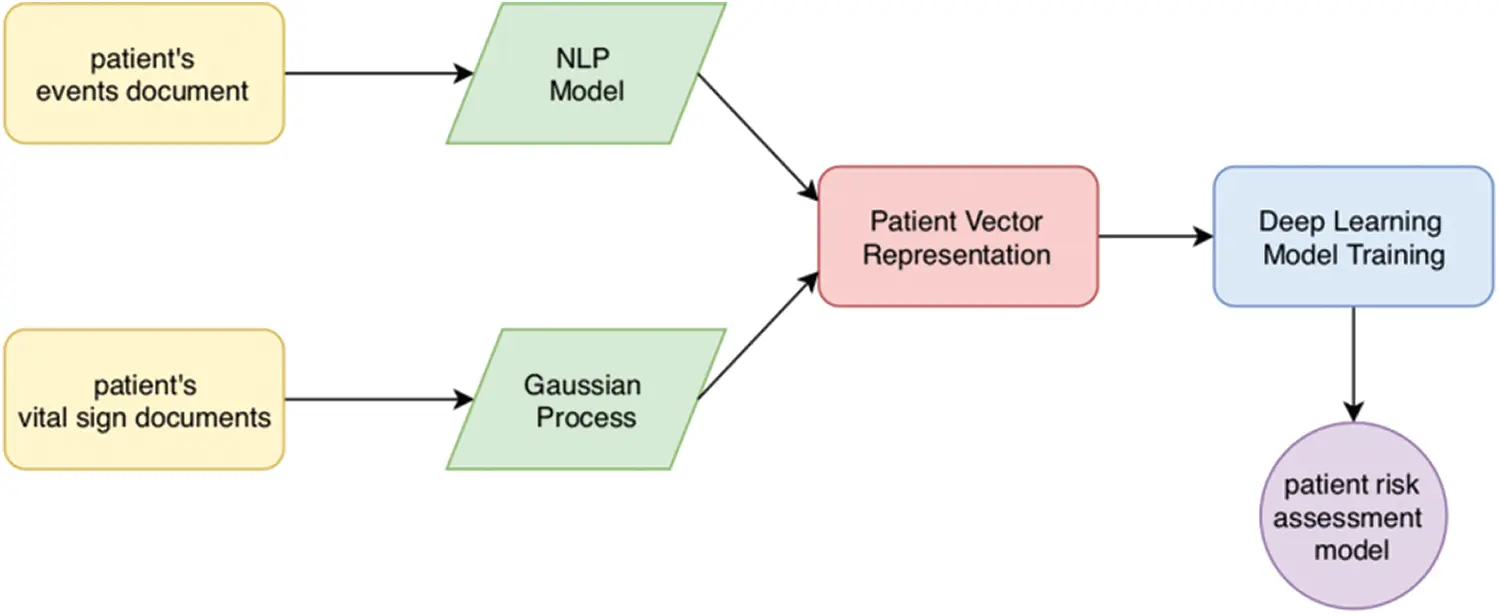Figure 1: Patient mortality risk assessment model pipeline

The process of preparing medical event representation for each patient is illustrated in Fig. 2. We represent each medication event using the context of patient’s medical record under the assumption that the target medical event and its context medical events are related. This kind of relationship can be captured using the NLP word vector representation technique. The related medical events should theoretically be close to each other in the embedding space constructed by the NLP word vector model. For example, Anticardiolipin Antibody is a fluid medicine in Hematology. Based on its context medical events, it should be close to Heparin, which is also a fluid medicine in Hematology and both of them are related to the treatment of blood clots. If two fluid medicine that are not semantically related to the treatment, one of the reasons could be human errors. The semantic relation inside EHR could be used to reduce the possibility of medical accidents.

We used the word2Vec model to construct word vector representation. word2Vec is a deep neural network model that can implement the vector representation of the word . In our design, illustrated in Fig. 3. We consider each event as a “word”, and all of these “words” construct the patient “document”. Then, all patients build up the entire corpus. There are two algorithms to train the word2Vec model: Continuous-Bag of Words (CBOW) and skip-gram. CBOW uses the target word to predict the context words, while skip-gram uses the context words to predict the target words. Both of the algorithms can be used for constructing the word vector representation. Each medical event can be represented as a dense vector, which is one of the input features of our model.

The process of building our own word2Vec model is very similar to the normal NLP process. Fig. 2 demonstrates the whole process of constructing vector representation of patient’s medical event and other numerical medical data in his/her EHR, and then concatenate together to be the input of the neural network.

Given a collection of medical events for patient i records Ei={Ei1,Ei2,Ei3,,Ein}, where n is the number of time points when events occur, and a set of vital signs and other numerical medical records, Ri={Ri1,Ri2,Ri3,,Rin}, where m is the number of time points when vital signs are recorded. After the word2Vec model, each medical event eR1b and the for each EijRn1(kb), where k is the time point that has the most medical events. Therefore the Ei has the dimension of EiRn1(kb). Similarity, the numerical medical records Ri has the dimension of RiRn1(ka), where m is the number of timestamps that have the records, a is the number of vital sign records. After the Gaussian Process, Ei and Ri will concatenate together to become the input tensor with dimension max(m,n)1(a+kb) for the network. We first do the stemming by removing all the information except the fluid related infusion item name ordered by time sequence. Those item names reconstruct each patient’s document and the entire corpus. Even though item names may consist of multiple English names, we still treat each item as a single word. Then, we build the dictionary, and our goal is to find the word vector. We trained our own word2Vec model based on this corpus using the CBOW algorithm. Then, an event ei can be represented as a word vector ei. Word vector events representation can distinguish different types of events. However, the difference between the same type of events cannot be captured. For the same type event that gives patients, the total dose amount, the infusion rate of the dose and the vital sign measurements account for the similarities of the events. Therefore, each event is represented as ei = <ei, total amount, rate, body temperature, pulse rate, respiration rate, blood pressure >, where the total amount, rate, and all the representative vital signs take into consideration of representing a medical event.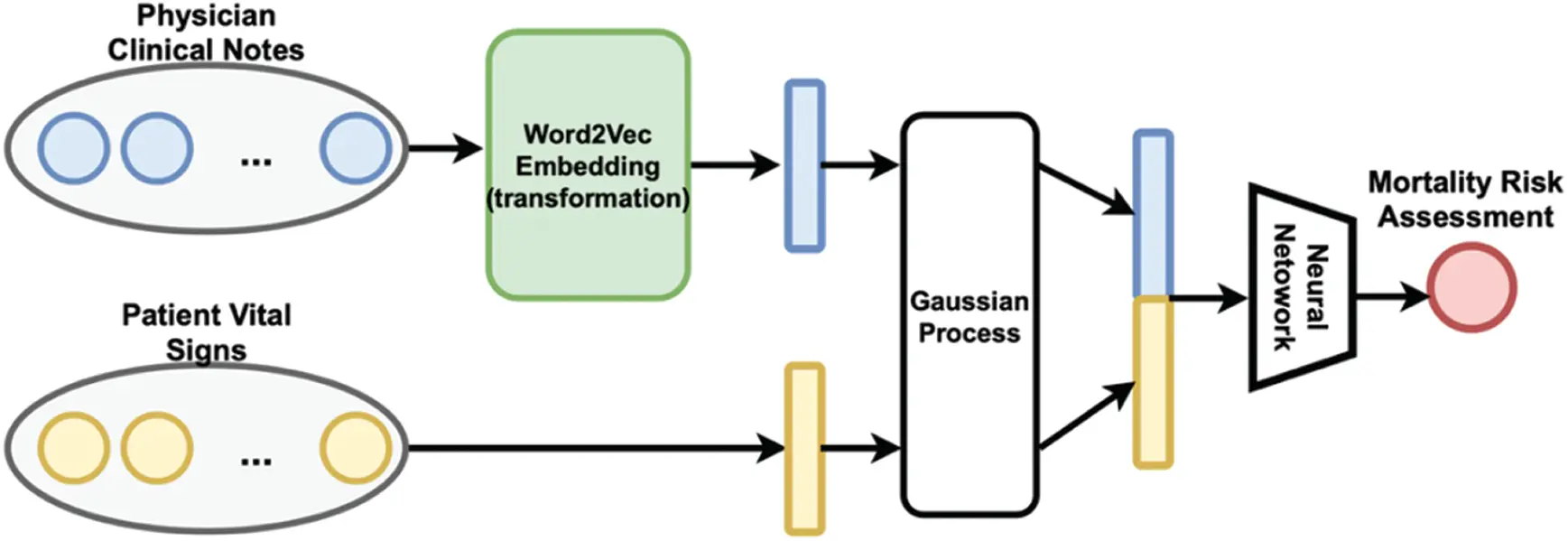Figure 2: The word2Vec model transformed patient’s clinical notes into vector representation

The Gaussian process imputes the missing values until all the values are uniformly distributed over time. Then, two vectors concatenate together as the input to the neural network. The dimension of the word vector may be too high and also increases the number of parameters in our model. The large number of parameters in the model could lead to problems like over-fitting, long training time, etc. We decided to apply the dimensional reduction technique on the word vector. We currently use the Principle Component Analysis (PCA) to reduce the dimension so that the length of event vector representation will be optimal for model input, while the similarities and dissimilarities of the event vector can still be preserved.

3.2 Gaussian Process Regression for Missing Values

The hallmarks of EHR data are the missing values, high dimensionality, irregular sampling and heterogeneous data types. These problems can greatly influence the performance of the prediction model. Fig. 3 illustrates the missing value and irregular sampling problems in a patient’s record. Some values are missing for some medical records and medical records may not be regularly distributed at patient’s timeline. One of the major problems in the medical data time series is the missing values of data caused by sparse data and irregular sampling. Gaussian process (GP) has been widely used for data imputation process  for time series data. It utilizes the “kernel trick”, Kernel function measures the similarities between observed samples, then imputes the missing value with the maximum likelihood.

This major problem in the time series of medical data is caused by sparse data and unbalanced sampling, which brings certain limitations to the application of model on mortality risk assessment task. Gaussian Process Regression is a machine learning method developed based on Bayesian theory and statistical learning theory. Its advantage is that the high predictive accuracy of data imputation can be achieved by a small number of hyper parameters.

EHR data is a time-series data that commonly has the missing-value and irregular sampling problem over time. We select Gaussian Process to pre-process the EHR data of each patient. Given a training set {X, Y}, Gaussian Process is able to predict a probability distribution of Y* over given X*. Thus, it can be used to do imputation for the “missed data”.

In Probability and Gaussian theory, the Gaussian process is a random process on the observations is a continuous variable. Here, we can assume that all the features about the patients’ medical records can be continuous and time-based random variable. The most important part of the Gaussian Process can be defined as its mean and kernel function.

{m(x)=E[f(x)]k(x,x)=E[(f(x)m(x))(f(x)m(x))] (1)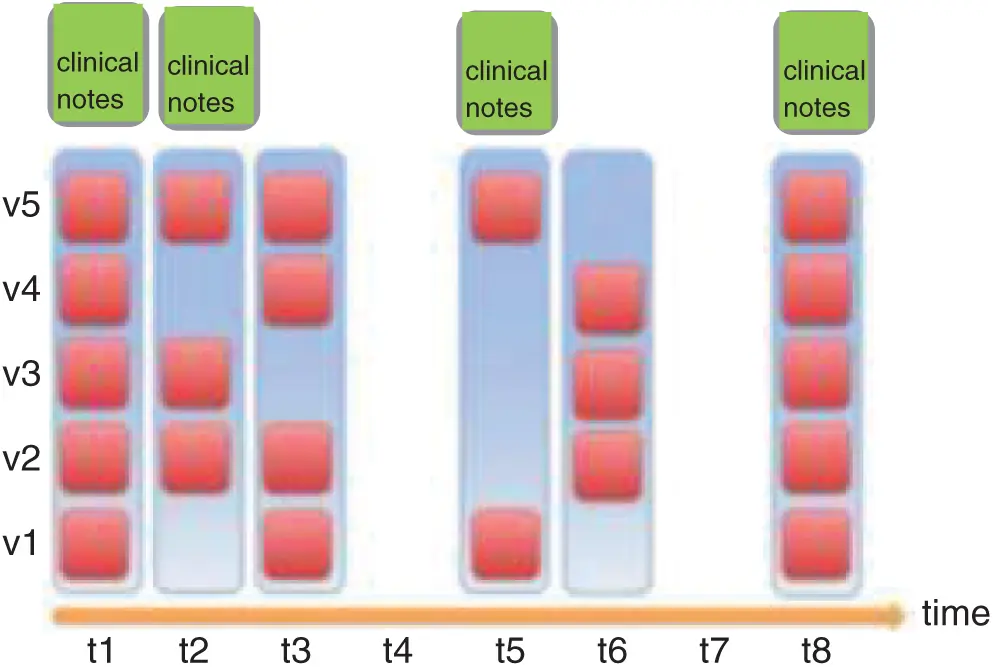Figure 3: An illustration of the problem: missing value, irregular sampling, and heterogeneous data types

Here, x,xRd is the arbitrary random variable. Therefore, Gaussian Process can be defined as:

f(x)GP(m(x),k(x,x)) (2)

As for data set {x, y} consists of n observations, its predicted value can be satisfied by the following model:

y=f(x)+ε (3)

The x is an input vector with the d dimension, y is the output vector. ε is the noise we added to the model by following the normal distribution, εN(0,σn2), its standard deviation is σn2. The output of y satisfies the distribution as illustrated below:

yN(m(x),k(x,x)+σn2In) (4)

Here, In is the identity matrix. When we usually preprocess the data and make it the mean function be 0. Based on the definition of Gaussian Process, the joint distribution of any finite random variables can also satisfy the Gaussian distribution.

Let x = {x1, x2, x3,…, xn} be the collection of patient’s event occurring time-stamp sequence from the patient record with n number of events, in particularly, we can denoted it as {f(xi): xi x}, which is the corresponding observed measurement value y, The predicted imputation value f and kernel function k(·,·). Then, the distribution of the set x is denoted as:

[yf]N(0,[k(x,x)+σn2Ink(x,x)k(x,x)k(x,x)]) (5)

The k(x,x) is the covariance matrix with the dimension of n by n, matrix element ki,j=k(xi,xj), k(x,x)=k(x,x)T is the covariance matrix between the predicted imputation x and the training input vector x that has the dimensionality of n by 1. k(x,x) is the covariance of the predicted imputation x. Hence, the posterior probability distribution f and variance σ(x) can be calculated as follows:

f|x,y,xN(f¯,σ(x)) (6)

f¯=k(x,x)[k(x,x)+σn2In]1y (7)

σ(x)=k(x,x)k(x,x)[k(x,x)+σn2]1k(x,x) (8)

where, the mean vector f¯ is the output of the Gaussian Process Regression model, so, the output value of the imputation point is:

f=m(x)+f¯ (9)

For the choice of the kernel function k(xi,xj), we select squared exponential (SE) covariance kernel function, which is defined as formula (8). The purpose of kernel function is to transform to a valid co-variance matrix corresponding to some multivariate Gaussian distribution while preserving the similarities between two observations. For a kernel transformation, the kernel function must satisfy the Mercer’s condition (illustrated in definition 1). In Mercer’s condition, the function needs to be square-integratable (illustrate in definition 2) Therefore, the squared exponential kernel is the commonly used kernel function, which is defined in Eq. (1), where parameter σf2 denotes the amplitude (y-scaling) and τ determines the smoothness of the Gaussian process prior with kSE(·,·).

Definition 1. Definition A real-valued kernel function K(x,y) satisfies Mercer’s condition if k(x,y)g(x)g(y)dxdy0 for all square-integrable functions g(·).

Definition 2. Definition A function g(x) is square-integrable if +|g(x)|2dx<

kSE(xi,xj)=σf2exp(12τ2xixj2) (10)

The squared exponential covariance function has only two hyper parameters, namely signal variance σf2 and length-scale τ. We used the maximum likelihood approach to find the appropriate initials value of them, and then apply the Newton method optimization approach during the model training, in order to find the best optimal values. First, we build the negative logarithm likelihood function L(θ).

L(θ)=12yTk1y+12log|k|+n2log(2π) (11)

The find the derivative with respective to the θi

L(θ)θi=12tr(αTαk1)kθi) (12)

where, k=kf+σn2In, α=k1y. Once we find the best optimal parameters on the training dataset, we use the formulas (7) and (8) to obtain the corresponding prediction value f and standard deviation σf2 for imputed value x.

Then, suppose we want to impute the missing value f(xk), we need to calculate the new co-variance matrix using the kernel function. We need to calculate the new vectors k*SE(xk, ·) and k*SE(xk, xk), where are

kSE(xk,)=[kSE(xk,x1)kSE(xk,x2)kSE(xk,xj)] (13)

And kSE(xk,xk)=[kSE(xk,xk)]

Then, the new co-variance matrix can be k(,)=kSE(xk,)Tk(,)kSE(xk,)+kSE(xk,xk). The imputed value can be the value calculated using the new co-variance matrix with maximum likelihood.

For each patient, the vector representation of the medical event has the missing-value problem on “rate”, “total amount”, and all the vital sign measurements. The “rate”, “total amount” are discrete variables associated with their medical events. For each medical event, we can simply use the above Gaussian Process to compute the max-likelihood values of “rate”, “total amount” for missing-value imputation. However, for the vital sign measurements, each medical event vector uses their mean values during the interval with the assumption that they must be uniformly distributed during the interval. In fact, all the vital measurements are irregularly sampled or even missing during the interval. We normalized the time stamps where this vital measurement was recorded and assumed the Using the Gaussian Process, we can impute the missing values for all vital sign measurements to make them regularly sampled and calculate the mean, illustrated in Figs. 4 and 5.

Data imputation is vital to the performance of our pipeline. For example, the missing value of the “rate” and “total amount” at time point Xi is likely to be affected/similar by Xj if these two time points are close to each other. However, a potential shortcoming of such method is that the computation workload could be heavy, especially when we want to build the model using more features. If one of the input feature dimension suffers irregularly-sampling or missing value problem, we need to build a new Gaussian Process Regressor. Finally, each patient will be represented as a fixed-length sequence of medical events with imputed data. Such sequence will be the input of our model.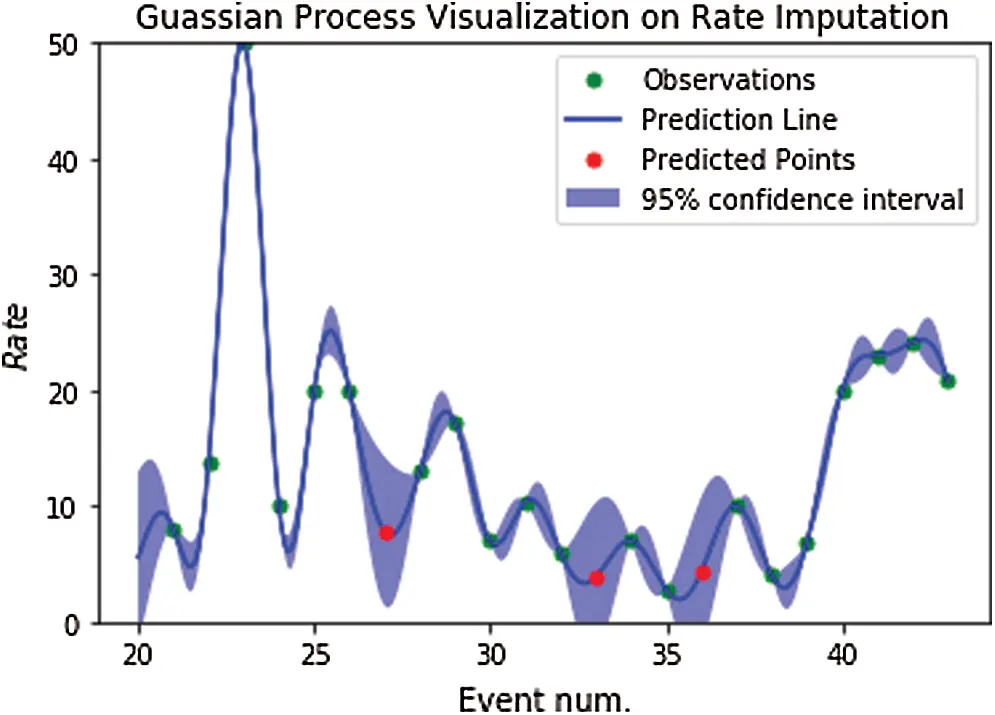Figure 4: Gaussian process effect visualization on rate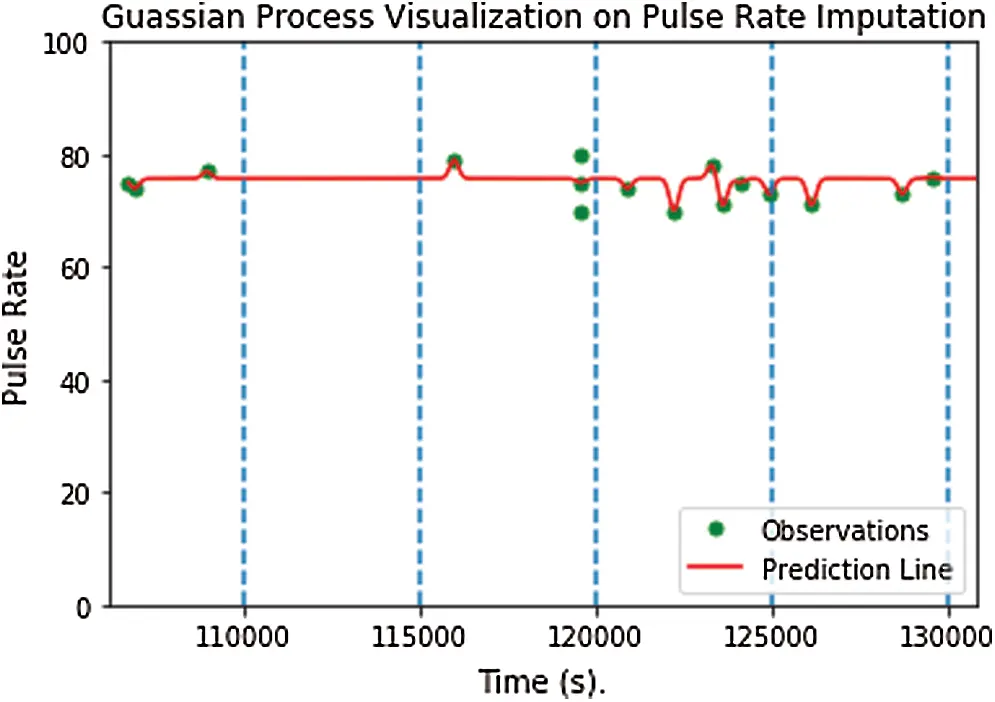Figure 5: Gaussian process effect visualization on pulse rate

4  Recurrent Neural Network Model

4.1 LSTM (Long Short Term Memory Recurrent Neural Network)

RNN based neural network is currently the state-of-the-art modeling method for sequential data. However, patients’ treatment process usually spams over a long period of time, and there is “vanishing gradient” problem. A variation of recurrent neural network, so called Long Short-Term Memory Unit (LSTM) has the better performance than RNN. The architecture of the LSTM can be viewed as a gated cell. The cell decides which information will be remembered or forgotten through gate opening and closing. By maintaining this gate switch, it allows LSTM to continue to learn over a long-time interval. There are three gate functions in LSTM neurons, namely input gate, output gate and forget gate.

it=σi(Wxixt+Whiht1+wcict1+bi) (14)

ft=σf(Wxfxt+Whfht1+wcfct1+bf) (15)

ct=ftct1+ittanh(Wxcxt+Whcht1+bc) (16)

σt=σo(Wxoxt+Whoht1+wcoct1+bo) (17)

ht=σttanh(ct) (18)

where, it,ft,ot respectively represents the input gate, output gate and forget gate function at time t. ct is the activation vector, xt and ht are the input vector at time t and the hidden layer output vector at time t. σi,σf,σh are sigmoidal nonlinearities and σc and σh are tanh nonlinearities. Wxi,Whi,Wxf,Whf,Wxc,Whc,Wxo,Who are the matrix parameters of the neural network. wci,wcf,wco,bo,bi,bf,bc are the vector parameters of the neural network. Among them, Wxi,Whi,Wxf,Whf,Wxo,Who are the weight parameters for different gates, with bias bo,bi,bf is element-wise (Hadamard) product. Since the LSTM decides to drop up some information at each time stamp, it is able to store the information from longer time stamp, when comparing with base-RNN.

4.2 Phased-LSTM

The increasing long term-dependency drives the researches focusing on improving the architecture of the LSTM cell. Based on the classic LSTM, in this paper, a time gate (phase gate) is designed for each hidden layer neuron. namely phased-LSTM. Of course, the structure of the neuron can be further improved, for example, add the filter gate, to improve the performance of the model. So, other than using regular LSTM, we also use phased LSTM  on modelling the outcome of patients’ treatment. The major difference between regular LSTM and phased LSTM is that phased LSTM can process irregularly sampled data that is caused by events in continuous time. Considering the data in EHR may trigger by irregular events, phased LSTM may have better performance on modeling our problem. Fig. 6 shows the architecture of Phased-LSTM cell.Figure 6: The architecture of Phased-LSTM cell

It adds a new time gate kt. The updates to neuron ct and ht can only be done when the gate is opened. In this way, the input can be periodically sampled to solve the problem of too long input sequence. The open and close of the gate is controlled by independent rhythm represented by three parameters. τ is controlling the time period of one open and close cycle. ron control the open phase duration ratio to the entire period. s controls the cycle shifts to each cell. All of these parameters are learned in the training processes. The follows are the formulas:

φ=(ts)modττ (19)

kt={2φtron,ifφt<12ron22φtron,if12ron<φt<ronαφt,otherwise (20)

φt indicates the different phases. The opening of the gate has two phases: the opening ratio is increasing from 0 to 1 on the 1st half of opening and decreases from 1 to 0 on the second half of the opening. When the gate is closed, there is a leaking rate α that can let important information go through even when the gate is closed, otherwise, there would be no information retained in the hidden layer.

This time gate allows the Phased-LSTM to solve the irregular sampling problem and also accelerate the training phrase. For example, the opening ratio can be large (close to 1) when the number of medication events inside the current interval is high; otherwise, and the opening ratio will adjust to a small value (close to 0). The number of medication events inside a time interval determines the value of the opening ratio and how much information will be updated to the output layer and hidden layer of Phased-LSTM cell.

The calculation of cj and hj are performed based on Eqs. (21)(24), and the previous ct and ht will be denoted as c*t and h*t.

ct=ftct1+ittanh(Wxcxt+Whcht1+bc) (21)

ct=ktct+(1kt)ct1 (22)

ht=ottanh(ct) (23)

ht=ktht+(1kt)ht1 (24)

Then, we defined the softmax layer that maps the outputs generated by the LSTM and Phased-LSTM cell into the probability representation using Eq. (25), where f(Cti) denotes as the probability of class i.

f(Cti)=expCtij|Ct|expCtj (25)

5  Experiment and Result

5.1 Date Sets

The experiments in this paper were carried out on death risk prediction data sets and clinical infusion drug event risk prediction data sets, which were generated from MIMIC III. We randomly split the whole data set into 2/3 training set and 1/3 test set. The MIMIC III database has a total of 46,520 (large number amount of patients’ hospitalization records) patients with fluid-related input records and vital sign records .

For fluid-related input records, we extracted the following information: (1) “itemid” is the identifier for a fluid related drug or substance. (2) “rate” lists the rate at which the drug or substance was administrated to the patient. (3) “total amount” lists the total amount of the fluid in the bag containing the solution. (4) “start time” and “end time” record the start and end time of an input/output event. This information will be used to build per-patient time-series event data.

For vital sign records, we extracted the most representative measurements as following: (1) body temperature: abnormal body temperature may be due to fever or hypothermia, or any adverse drug effect. (2) pulse rate: the pulse rate can be included as heart rhythm and the strength of the pulse. (3) respiration rate: fever, illness, or other medical conditions may cause the abnormal respiration rate. (4) blood pressure: the blood pressure can be categorized into 4 stages: normal, elevated, Stage 1 and Stage 2, which reflect the condition of the heart. All of the above measurements, the “rate”, and “total amount” are continuous numerical values and suffer the missing values and irregular sampling.

5.2 Experimental Results and Analysis

5.2.1 Model Architecture

We built the same architecture of LSTM and Phased-LSTM model (Figs. 7 and 8): 106 feature inputs, 309 hidden units with 2 layers. The number of layers and features we selected are based on the experience and the dataset. At the output of the network, we add a softmax layer that is used for the classification task. Phased-LSTM deep neural network requires another dimension of input feature: time. We arranged the medical events in the order of their occurring time and trained LSTM based model and phased-LSTM model. We implemented the LSTM model and Phased-LSTM using Theano.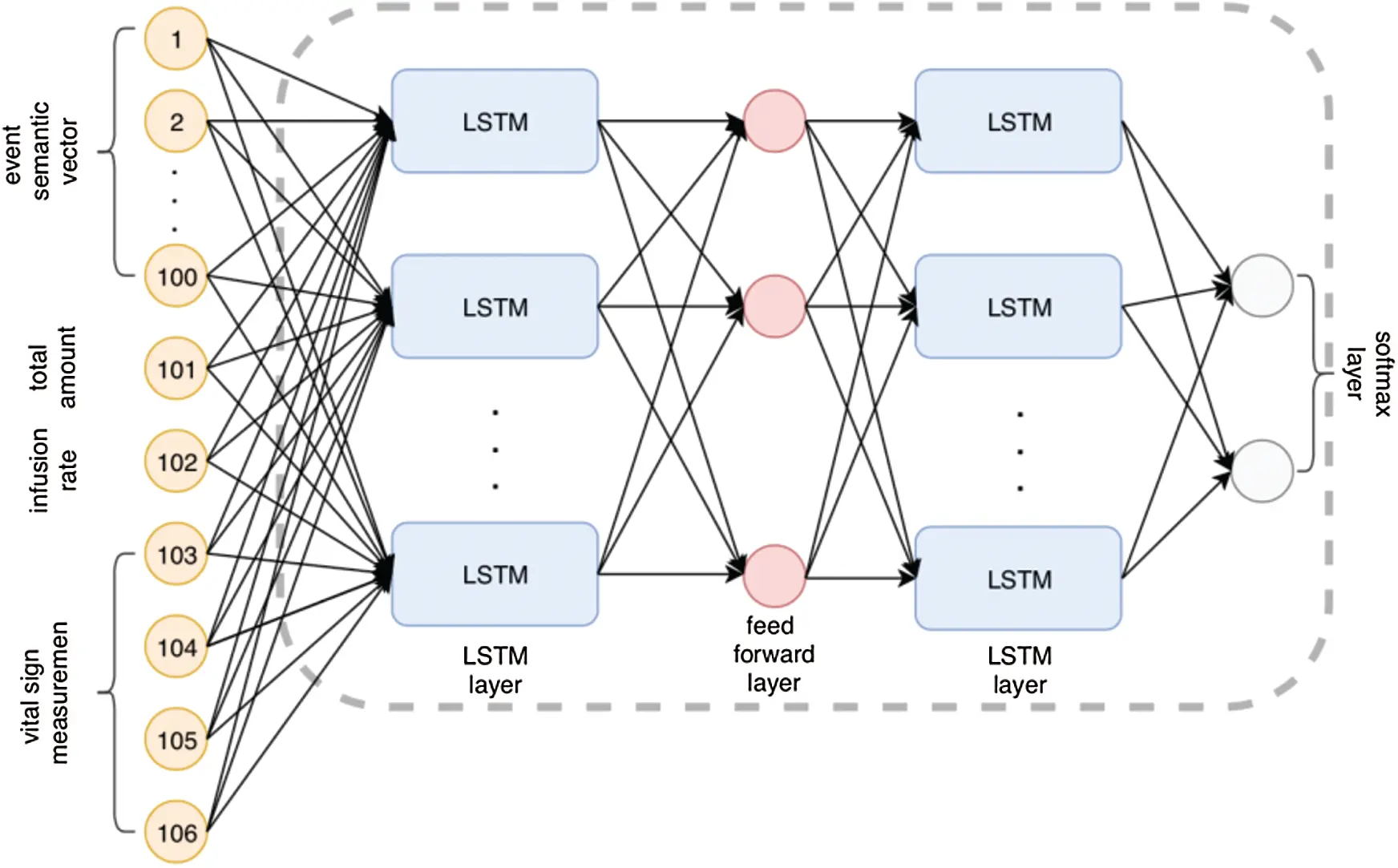Figure 7: The architecture design of LSTM based modelFigure 8: The architecture design of Phased-LSTM based model

The choice of optimization and loss function are determined by the task of our models and the dataset itself. We compared several loss functions and optimizers. The Cross-Entropy loss and Adaptive Momentum Optimization (Adam) optimizer gave the model the best output.

The output of the model is two probabilities: [Prob(survival), 1-Prob(survival)/prob(dead)], denoted as [p(v1), p(v2)]. If p(v1) > p (v2), then we classify the patient as survival (1), otherwise, we classify the patient as dead (0). We turned this mortality prediction as a binary problem. We used the binary cross entropy (BEC) as the loss measurement of the model.

Eq. (26) is the mathematical expression of the binary cross entropy measurement of our model at each data sample, where N is the number of samples. The model uses the loss during the learning phase to gradually adjust the model until there is no improvement or very small improvement.

Loss=1Ni=1Nyilogy^i+(1yi)log(1y^i) (26)

Figs. 9 and 10 shows the loss of the LSTM and Phased-LSTM at each epoch. The epoch defines the training phase of LTSM and Phased-LSTM. The loss of the model is calculated by the MSE mathematical function and it indicates the model’s earning outcomes. Both of the models aim to minimize the loss value during the training. At the initial, the loss of LSTM is lower than Phased-LSTM; then after around 10 epochs, the loss of Phased-LSTM can dramatically reduce its loss value (converge at a faster rate). The reason is because of the nature of the Phased-LSTM itself. The close and open of the time gate of the Phased-LSTM prevents the information from entering into the cell’s memory, which causes that the loss of Phased-LSTM is higher than LSTM. However, the Phased-LSTM can converge at a stunning rate, which decides is rapidly reducing its loss during the initial phase of the training. This indicates another advantage of Phased-LSTM for fast converging.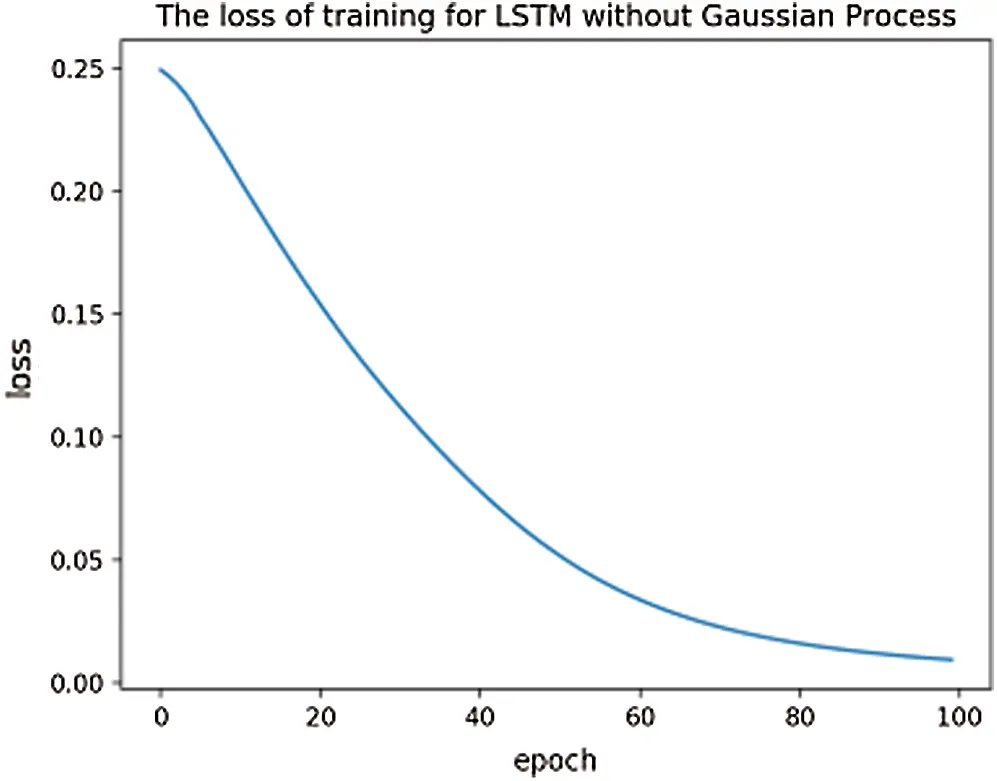Figure 9: The training loss of LSTM: epoch = 100, learning rate = 0.01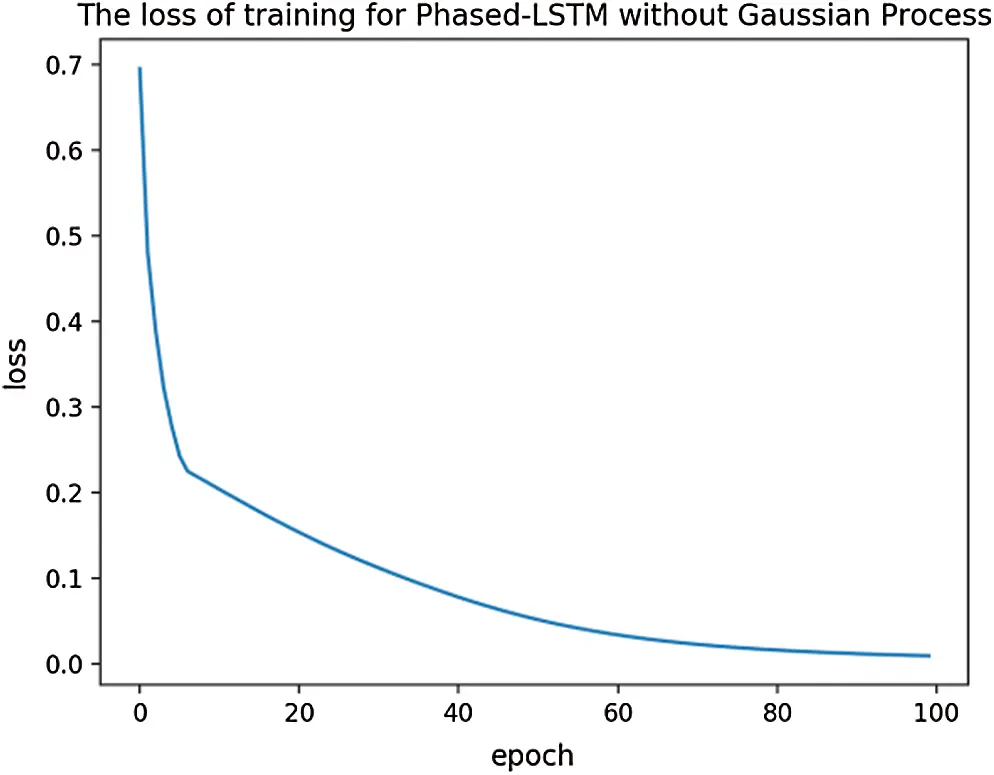Figure 10: The training loss of Phased-LSTM: epoch = 100, learning rate = 0.01

5.2.2 Model Performance

We first investigate the impact of using Gaussian Process for data imputation on models’ performance to ensure the effectiveness of Guasisan Process and Phased-LSTM indeed can improve dataset and the model performance. During the evaluation of the model performance, we compare the different data imputation approaches that are suitable for time-series missing values imputation. We assume the EHR data are missing random (MAR), which is usually defined as the pattern for a variable is not a function of its observed values because the patient’s medical readings are stochastically changing. The first step is to deal with heterogenous data types by combining the paitent’s numerical information such as vital sign information, drug amount and textual information fluid related medical events such as drug names. Then, we compared several different data imputation approaches: mean imputation (baseline), Autoregressive with exogenous inputs (ARX), Auotoregressive moving average model (ARMA), Autoregressive integrated moving average (ARIMA), and Gaussian Process (GP) for improving the dataset. Next, we compare the results obtained by LSTM model and Phased-LSTM. Finally, we compare the proposed models with other machine learning algorithms. In order to show the Phased-LSTM tackles down the long sequence data, we filter out patients with a small number of medical events and construct the training and testing dataset with the appropriate number of patient instances.

We use ROC curve, precision and recall score to evaluate the model’s performance. The experiment results show the comparison among the LSTM model and Phased-LSTM model with and without the Gaussian Process. Some experiment results are shown in Figs. 11 and 12 and Tab. 1.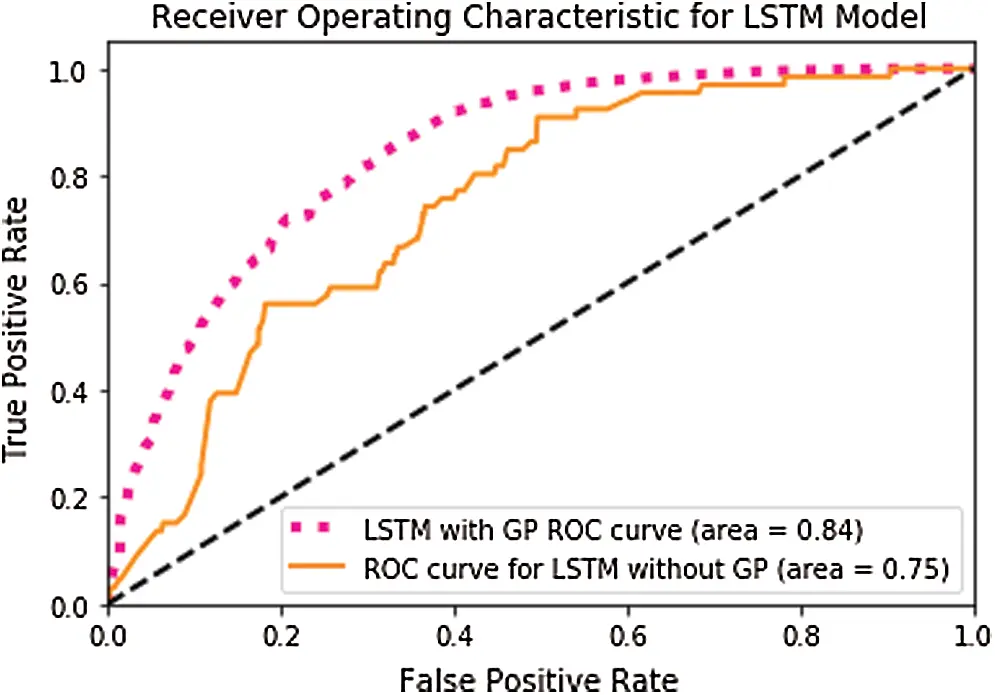Figure 11: The ROC curve for LSTM model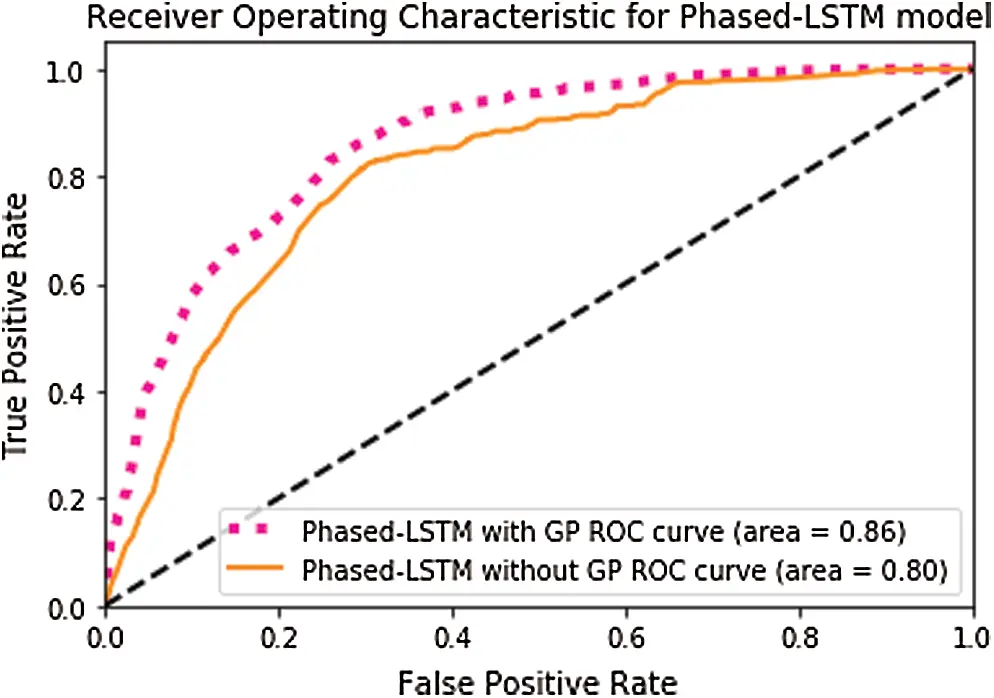Figure 12: The ROC curve for Phased-LSTM model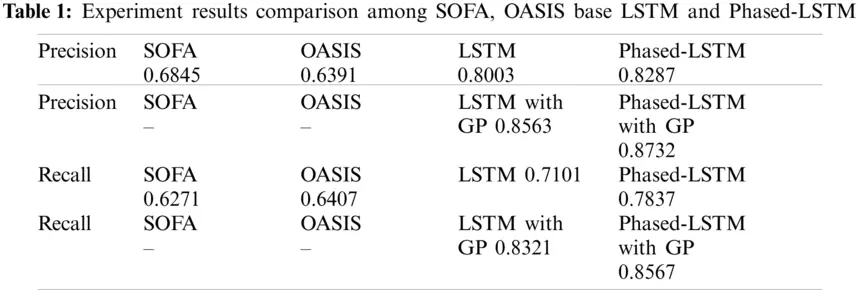From Figs. 11 and 12 and Tab. 1, first, the models greatly outperform the original method. We concentrate on comparing the performance of deep learning models with the traditional physical approaches: SOFA and OASIS. We treated SOFA and OASIS as the benchmark results and LSTM as the baseline. We are mainly interested in the comparison between LSTM and Phased-LSTM model and the effect of the Gaussian process. The experimental results indicate that our hypothesis of phased-LSTM is better being applied to solving challenges in ICU data. The Phased-LSTM achieved the highest performance among all other methods. Second, we can see that models with Gaussian Process have higher precision and recall scores and ROC curve. This is due to the serious problem of missing values inside the health care dataset. The model’s performance will be impaired if we do not decide to impute them. However, models with Gaussian Process also introduce another problem that is the training time. For each patient instance, we need to train its own GP regressor then construct the input for the model. Furthermore, the Phased-LSTM model is also able to give better results as we expected because each patient instance usually has the long temporal dependency.

The Tab. 2 shows the different imputation methods for time series data and Phased-LSTM network. The results indicate the GP achieved comparatively better performance compared with others, but we care more about precision value because of the application of mortality prediction. Since the Mean and ARX methods are more suitable for data with linear relationships, especially for ARX models, they are designed for a dynamic system in discrete time. The ARMA and ARIMA share several similarities. The main difference is that ARMA is a stationary model. The “integrated” property of ARIMA allows the model to measure the non-seasonal differences needed to achieve the stationarity. If there are no such differences, then ARMA and ARIMA are the same. However, the GP is great for modeling the uncertainty, which is common inside the EHR dataset.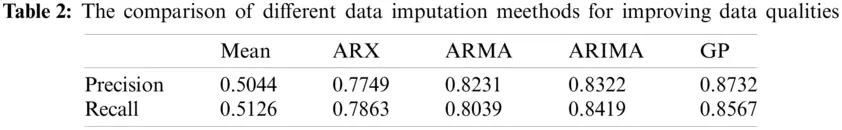6  Conclusion

Missing values, irregular sampling, heterogeneous data types, high dimensionality and long temporal dependency contribute to the difficulty of analysis of health care data, especially in ICU environment. We proposed a data-preprocessing pipeline using statistical approach and natural language processing technique. In addition, we used a new LSTM type called Phased-LSTM to deal with irregular sampling and long temporal dependency inside the data. The experiments show that using the Phased-LSTM framework with the proposed preprocessing pipeline indeed can give us the promising results in the mortality prediction task. Our future work plans to apply our pipeline and model on more complex data by not only including the fluid related medical events. We will also add more vita sign data, other medication events and important device management data. It is hoped that the model can predict risks more accurately, evaluate clinical medication events, and automate the management of important equipment. We also empirically compared with different data imputation methods for improving the HER time series dataset.

Our future work will focus on improving the prediction accuracy of our approach in a real ICU environment by trying different prediction networks and data imputation approaches. In addition, we will also try the neural network based approach for time series imputation such as Bidirectional Recurrent network and End-to-End Generative Adversarial Network (E2GAN).

Funding Statement: This research is supported by Natural Science Foundation of Hunan Province (No. 2019JJ40145), Scientific Research Key Project of Hunan Education Department (No. 19A273) and Open Fund of Key Laboratory of Hunan Province (2017TP1026).

Conflicts of Interest: The authors declare that they have no conflicts of interest to report regarding the present study.

## References

1. Soni, S. R., Khunteta, A., Gupta, M. (2011). A review on intelligent methods used in medicine and life science. Proceedings of the International Conference & Workshop on Emerging Trends in Technology, pp. 703–706. New York, NY, USA.
2. Hussain, A. (2017). Machine learning approaches for extracting genetic medical data information. Proceedings of the Second International Conference on Internet of things, Data and Cloud Computing, pp. 1. New York, NY, USA.
3. Bhattacharya, S., Rajan, V., Shrivastava, H. (2017). ICU mortality prediction: A classification algorithm for imbalanced datasets. Proceedings of the AAAI Conference on Artificial Intelligence, pp. 31. San Francisco, CA, USA.
4. Agniel, D., Kohane, I. S., & Weber, G. M. (2018). Biases in electronic health record data due to processes within the healthcare system: Retrospective observational study. BMJ, 361, k1479. [Google Scholar] [CrossRef]
5. Baek, H., Cho, M., Kim, S., Hwang, H., & Song, M. (2018). Analysis of length of hospital stay using electronic health records: A statistical and data mining approach. PLos One, 13(2), 1-16. [Google Scholar] [CrossRef]
6. Ribas, V. J., Lpez, J. C., Ruiz-Rodrguez, J. C., Ruiz-Sanmartn, A., Rello, J. et al. (2011). On the use of decision trees for ICU outcome prediction in sepsis patients treated with statins. IEEE Symposium on Computational Intelligence and Data Mining (CIDM), pp. 37–43. Paris, France.
7. Nachimuthu, S., Wong, A., Haug, P. (2010). Modeling glucose homeostasis and insulin dosing in an intensive care unit using dynamic bayesian networks. AMIA Annual Symposium Proceedings/AMIA Symposium, pp. 532–536. Washington DC, USA.
8. Wang, S. L., Wu, F., & Wang, B. H. (2010). Prediction of severe sepsis using svm model. Advances in Experimental Medicine and Biology, 680, 75-81. [Google Scholar] [CrossRef]
9. Nie, L., Wang, M., Zhang, L., Yan, S., & Zhang, B. (2015). Disease inference from health-related questions via sparse deep learning. IEEE Transactions on Knowledge and Data Engineering, 27(8), 2107-2119. [Google Scholar] [CrossRef]
10. Rav, D., Wong, C., Deligianni, F., Berthelot, M., & Andreu-Perez, J. (2017). Deep learning for health informatics. IEEE Journal of Biomedical and Health Informatics, 21(1), 4-21. [Google Scholar] [CrossRef]
11. Lv, X., Guan, Y., Yang, J., & Wu, J. (2016). Clinical relation extraction with deep learning. International Journal of Hybrid Information Technology, 9(7), 237-248. [Google Scholar] [CrossRef]
12. He, K., Zhang, X., Ren, S., Sun, J. (2016). Deep residual learning for image recognition. 2016 IEEE Conference on Computer Vision and Pattern Recognition (CVPR), pp. 770–778, Las Vegas, NV. DOI 10.1109/CVPR.2016.90. [CrossRef]
13. Rajkomar, A., Oren, E., Chen, K., Dai, A. M., & Hajaj, N. (2018). Scalable and accurate deep learning with electronic health records. Digital Medicine, 1(1), 1-10. [Google Scholar] [CrossRef]
14. Blunsom, P., Cho, K., Dyer, C., & Schütze, H. (2017). From characters to understanding natural language (C2NLU): Robust end-to-end deep learning for NLP (Dagstuhl Seminar 17042). Dagstuhl Reports: Vol. 7, pp. 129-157. Dagstuhl, Germany.
15. Johnson, A., Pollard, T., Shen, L., Lehman, L. W., & Feng, M. (2016). Mimic-III, a freely accessible critical care database. Scientific Data, 3(1), 160035. [Google Scholar] [CrossRef]
16. Liu, L., Shen, J., Zhang, M., Wang, Z., & Li, H. (2019). Deep learning based patient representation learning framework of heterogeneous temporal events data. Big Data, 1(1), 25-38. [Google Scholar] [CrossRef]
17. Hochreiter, S., & Schmidhuber, J. (1997). Long short-term memory. Neural Computation, 9(8), 1735-1780. [Google Scholar] [CrossRef]
18. Neil, D., Pfeiffer, M., & Liu, S. C. (2016). Phased lstm: Accelerating recurrent network training for long or event-based sequences. Advances in Neural Information Processing Systems, 29, 3882-3890. [Google Scholar]
19. Zhang, Y., Li, P., Zhao, X., Xia, E., Mei, J. et al. (2019). Predicting prevalence of respiratory disease with multi-task gaussian process: A case study in East China. 2019 IEEE International Conference on Healthcare Informatice (ICHI), pp. 1–3. Xian, China. DOI 10.1109/ICHI.2019.8904849. [CrossRef]
20. Caballero Barajas, K. L., Akella, R. (2015). Dynamically modeling patient’s health state from electronic medical records: A time series approach. Proceedings of the 21th ACM SIGKDD International Conference on Knowledge Discovery and Data Mining, pp. 69–78. New York, NY, USA, ACM.
21. Krompass, D., Esteban, C., Tresp, V., Sedlmayr, M., & Ganslandt, T. (2014). Exploiting latent embeddings of nominal clinical data for predicting hospital readmission. KI-Künstliche Intelligenz, 29(2), 153-159. [Google Scholar] [CrossRef]
22. Jonnagaddala, J., Dai, H. J., Ray, P., & Liaw, S. T. (2015). A preliminary study on automatic identification of patient smoking status in unstructured electronic health records. Proceedings of BioNLP, 15, 147-151. [Google Scholar] [CrossRef]
23. Pyysalo, S., Ginter, F., Moen, H., Salakoski, T., Ananiadou, S. (2013). Distributional semantics resources for biomedical text processing. Proceedings of LBM 2013, pp. 39–44. Tokyo, Japan.
24. Mikolov, T., Chen, K., Corrado, G., Dean, J. (2013). Ecient estimation of word representations in vector space. ICLR 2013, Scottsdale, Arizona, USA. arXiv: 1301.3781.
25. Wang, M., Niu, S. Z., & Gao, Z. G. (2019). A novel scene text recognition method based on deep learning. Computers, Materials & Continua, 60(2), 781-794. [Google Scholar] [CrossRef]
26. Pennington, J., Socher, R., & Manning, C. D. (2014). Glove: Global vectors for word representation. EMNLP, 14, 1532-1543. [Google Scholar] [CrossRef]
27. Bojanowski, P., Grave, E., Joulin, A., & Mikolov, T. (2017). Enriching word vectors with subword information. Transactions of the Association for Computational Linguistics, 5(1), 135-146. [Google Scholar] [CrossRef]
28. Joulin, A., Grave, E., Bojanowski, P., Mikolov, T. (2017). Bag of tricks for efficient text classification. Proceedings of the 15th Conference of the European Chapter of the Association for Computational Linguistics, vol. 2, pp. 427–431. Valencia, Spain.
29. Krishnan, G. S., Kamath, S. S. (2019). Evaluating the quality of word representation models for unstructured clinical text based ICU mortality prediction. Proceedings of the 20th International Conference on Distributed Computing and Networking, pp. 480–485. New York, NY, USA.
30. Xu, F., Zhang, X. F., Xin, Z. H., & Yang, A. (2019). Investigation on the Chinese text sentiment analysis based on convolutional neural networks in deep learning. Computers, Materials & Continua, 8(3), 697-709. [Google Scholar] [CrossRef]
31. Wu, H., Liu, Q., & Liu, X. D. (2019). A review on deep learning approaches to image classification and object segmentation. Computers, Materials & Continua, 60(2), 575-597. [Google Scholar] [CrossRef]
32. Chan, K. R., Lou, X., Karaletsos, T., Crosbie, C., Gardos, S. M. et al. (2013). An empirical analysis of topic modeling for mining cancer clinical notes. IEEE 13th International Conference on Data Mining Workshops, pp. 56–63. Dallas, TX, USA.
33. Reddy, C. (2015). Simultaneous discovery of common and discriminative topics via joint nonnegative matrix factorization. Proceedings of the 21th ACM SIGKDD International Conference on Knowledge Discovery and Data Mining, pp. 567–576. New York, NY, USA.
34. Titterington, D., & Sedransk, J. (1989). Imputation of missing values using density estimation. Statistics Probability Letters, 8(5), 411-418. [Google Scholar] [CrossRef]
35. Gulati, A., & Ferguson, R. J. (2009). Nfft: Algorithm for irregular sampling. CREWES Research Report, 21, 1-19. [Google Scholar]
36. Chen, N., Qian, Z., Nabney, I. T., & Meng, X. (2014). Wind power forecasts using gaussian processes and numerical weather prediction. IEEE Transactions on Power Systems, 29(2), 656-665. [Google Scholar] [CrossRef]
37. Rasmussen, C. E., Williams, C. K. I. (2005). Gaussian Processes for Machine Learning (Adaptive Computation and Machine Learning). USA: The MIT Press.
38. Hochreiter, S. (1998). The vanishing gradient problem during learning recurrent neural nets and problem solutions. International Journal of Uncertainty, Fuzziness and Knowledge-Based Systems, 6(2), 107-116. [Google Scholar] [CrossRef]
39. Koutnik, J., Greff, K., Gomez, F., Schmidhuber, J. (2014). A clockwork rnn. Proceedings of the 31st International Conference on Machine Learning, vol. 32, pp. 1863–1871. Beijing, China.
40. Mikolov, T., Chen, K., Corrado, G., Dean, J. (2013). Efficient estimation of word representations in vector space. ICLR Workshop, Scottsdale, AZ, USA. arXiv: 1301.3781v1.
41. Wolff, T. D., Cuevas, A., Tobar, F. (2020). Gaussian process imputation of multiple financial series. IEEE International Conference on Acoustics, Speech and Signal Processing, pp. 8444–8448. Virtual Barcelona, Spain.
42. Rodrigues, F., Henrickson, K., & Pereira, F. C. (2019). Multi-output gaussian processes for crowdsourced traffic data imputation. IEEE Transactions on Intelligent Transportation Systems, 20(2), 594-603. [Google Scholar] [CrossRef]
43. Nickerson, P., Baharloo, R., Davoudi, A., Bihorac, A., Rashidi, P. (2018). Comparison of Gaussian processes methods to linear methods for imputation of sparse physiological time series. Annual International Conference of the IEEE Engineering in Medicine and Biology Society, pp. 4106–4109. Honolulu, HI, USA.This work is licensed under a Creative Commons Attribution 4.0 International License, which permits unrestricted use, distribution, and reproduction in any medium, provided the original work is properly cited.